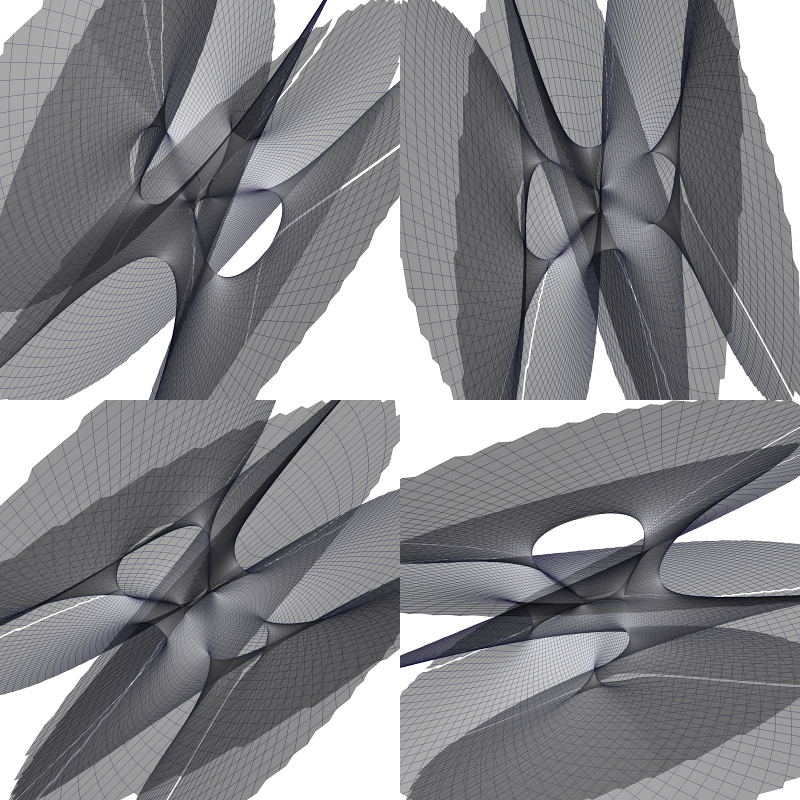# Bolza surface

Bolza surface300px|right|thumb">
Perspective projection of $y^2=x^5-x$ in $mathbb C^2$.In mathematics, the Bolza surface is a compact Riemann surface of genus 2 with the highest possible order of the conformal automorphism group in this genus, namely 48. An affine model for the Bolza surface can be obtained as the locus of the equation

:$y^2=x^5-x$

in $mathbb C^2$. Of all genus 2 hyperbolic surfaces, the Bolza surface has the highest systole. As a hyperelliptic surface, it arises as the ramified double cover of the 2-sphere, with ramification locus at the six vertices of a regular octahedron inscribed in the sphere, as can be readily seen from the equation above.

Triangle surface

The Bolza surface is a (2,3,8) triangle surface. More specifically, the Fuchsian group defining the Bolza surface is a subgroup of the group generated by reflections in the sides of a hyperbolic triangle with angles $frac\left\{pi\right\}\left\{2\right\}, frac\left\{pi\right\}\left\{3\right\}, frac\left\{pi\right\}\left\{8\right\}$. More specifically, it is a subgroup of the index-two subgroup of the group of reflections, which consists of products of an even number of reflections, which has an abstract presentation in terms of generators $s_2, s_3, s_8$ and relations $s_2\left\{\right\}^2=s_3\left\{\right\}^3=s_8\left\{\right\}^8=1$ as well as $s_2 s_3 = s_8$. The Fuchsian group defining the Bolza surface is also a subgroup of the (3,3,4) triangle group, which is a subgroup of index 2 in the (2,3,8) triangle group. It is interesting to note that the (2,3,8) group does not have a realisation in terms of a quaternion algebra, but the (3,3,4) group does.

Quaternion algebra

Following MacLachlan and Reid, the quaternion algebra can be taken to be the algebra over $mathbb\left\{Q\right\}\left(sqrt\left\{2\right\}\right)$ generated as an associative algebra by generators "i,j" and relations :$i^2=-3,;j^2=sqrt\left\{2\right\},;ij=-ji,$

with an appropriate choice of an order.

ee also

*Klein quartic
*Macbeath surface

References

* Katz, M.; Sabourau, S.: An optimal systolic inequality for CAT(0) metrics in genus two. Pacific J. Math. 227 (2006), no. 1, 95-107. See arXiv|math.DG|0501017.

* Maclachlan, C.; Reid, A.: The Arithmetic of Hyperbolic 3-Manifolds. Graduate Texts in Math., 219. Springer, 2003.

Wikimedia Foundation. 2010.

Поможем сделать НИР

### Look at other dictionaries:

• Surface de Bolza — Représentation en perspective de l équation y2 = x5 − x dans . En mathématiques, la surface de Bolza (du nom d Oskar Bolza) est une surface de Riemann compacte de g …   Wikipédia en Français

• Oskar Bolza — (1857 1942) Born 12 May 1857(1857 05 12 …   Wikipedia

• Riemann surface — For the Riemann surface of a subring of a field, see Zariski–Riemann space. Riemann surface for the function ƒ(z) = √z. The two horizontal axes represent the real and imaginary parts of z, while the vertical axis represents the real… …   Wikipedia

• Genus-2 surface — A genus 2 surface. In mathematics, a genus 2 surface (also known as a double torus or two holed torus) is a surface formed by the connected sum of two tori. That is to say, from each of two tori the interior of a disk is removed, and the… …   Wikipedia

• Octahedral symmetry — The cube is the most common shape with octahedral symmetry A regular octahedron has 24 rotational (or orientation preserving) symmetries, and a symmetry order of 48 including transformations that combine a reflection and a rotation. A cube has… …   Wikipedia

• Differential geometry of surfaces — Carl Friedrich Gauss in 1828 In mathematics, the differential geometry of surfaces deals with smooth surfaces with various additional structures, most often, a Riemannian metric. Surfaces have been extensively studied from various perspectives:… …   Wikipedia

• Projet:Mathématiques/Liste des articles de mathématiques — Cette page n est plus mise à jour depuis l arrêt de DumZiBoT. Pour demander sa remise en service, faire une requête sur WP:RBOT Cette page recense les articles relatifs aux mathématiques, qui sont liés aux portails de mathématiques, géométrie ou… …   Wikipédia en Français

• Systolic geometry — In mathematics, systolic geometry is the study of systolic invariants of manifolds and polyhedra, as initially conceived by Charles Loewner, and developed by Mikhail Gromov and others, in its arithmetic, ergodic, and topological manifestations.… …   Wikipedia

• List of differential geometry topics — This is a list of differential geometry topics. See also glossary of differential and metric geometry and list of Lie group topics. Contents 1 Differential geometry of curves and surfaces 1.1 Differential geometry of curves 1.2 Differential… …   Wikipedia

• List of mathematics articles (B) — NOTOC B B spline B* algebra B* search algorithm B,C,K,W system BA model Ba space Babuška Lax Milgram theorem Baby Monster group Baby step giant step Babylonian mathematics Babylonian numerals Bach tensor Bach s algorithm Bachmann–Howard ordinal… …   Wikipedia